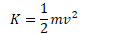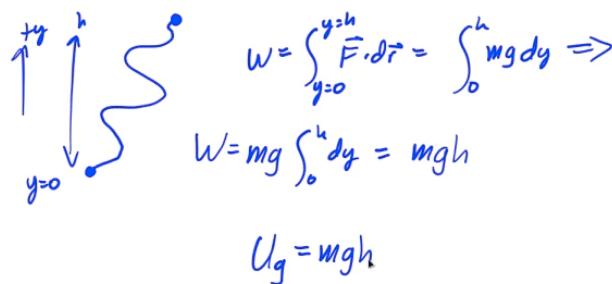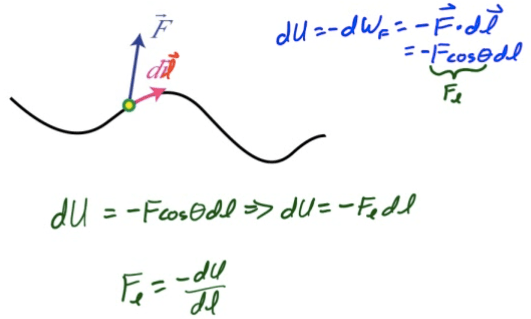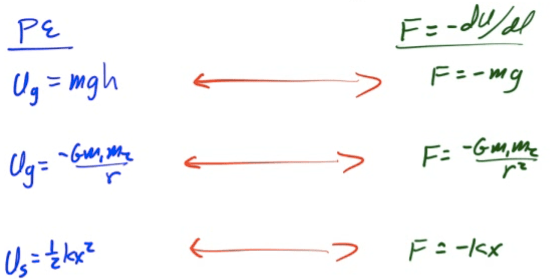# What is Energy?

• Energy is the ability or capacity to do work

• Work is the process of moving an object
• Energy is the ability or capacity to move an object

# Energy Transformations

• Energy can be transformed from one type to another

• You can transfer energy from one object to another by doing work

• Work-Energy Theorem

• Work done on a system by an external force changes the energy of the system

# Kinetic Energy

• Kinetic Energy is energy of motion

• The ability or capacity of a moving object to move another object
•# Potential Energy

• Potential Energy (U) is energy an object possesses due to its position or state of being

• Gravitational Potential Energy (Ug) is the energy an object possesses because of its position in a gravitational field

• Elastic Potential Energy (Us)

• Chemical Potential Energy

• Electric Potential Energy

• Nuclear Potential Energy

• A single object can have only kinetic energy, as potential energy requires an interaction between objects

# Internal Energy

• The internal energy of a system include the kinetic energy of the objects that make up the system and the potential energy of the configuration of the objects that make up the system

• Changes in a system's structure can result in changes in internal energy

# Gravitational Potential Energy (Ug)# Conservative Forces

• A force in which the work done on an object is independent of the path taken is known as conservative force

• A force in which the work done moving along a closed path is zero

• A force in which the work done is directly related to a negative change in potential energy (W=-ΔU)

Conservative Forces Non-Conservative Forces
Gravity
Elastic Forces
Coulombic Forces
Friction
Drag
Air Resistance

# Newton's Law of Universal Gravitation

• The gravitational force of attraction between any two objects with mass

•# Force from Potential Energy# Summary